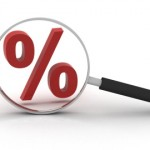Percentage Calculator

Free Percentage Calculator

 What is % of ? Answer: Or... is what percent of ? Answer: %

Percentage Calculator – PercentageA percentage is a method of expressing an amount as a fraction of 100. It is frequently represented by the percent sign, “%”, or the shortening “pct.”. For example, 57% is equal to 57 / 100, or 0.57.
Percentages are used to precise how big/small one amount is, comparative to another amount. The first amount typically symbolizes a part of, or a change in, the second amount, which must be bigger than zero. For example, a rise of \$ 0.15 on a value of \$ 2.50 is an increase by a fraction of 0.15 / 2.50 = 0.06. In a percentage, this is therefore a 6% rise.

Percentage Calculator – About

Percentage calculator will help you in two ways.

Responding: “What is X%  of Y ?” and “X is what percent of Y ?